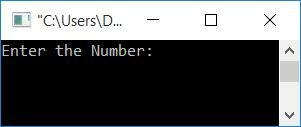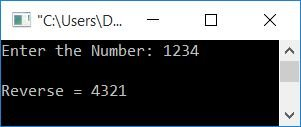# C Program to Reverse a Number

In this article, you will learn and get code for reversing a given number by the user at run-time in the following ways:

### How does the inverse of a number get calculated?

The very basic things to do to reverse a number are:

• Find the remainder of that number, one by one, until the number becomes equal to 0.
• While finding the remainder, initialize the new value to a variable that holds the reverse of the number.
• Every time while initializing the new value to the rev variable (that holds the reverse of the given number), don't forget to multiply 10 with its previous value and add the remainder.

For example, let's suppose a user enters a number, say 1234 (initialized to the num variable). Then here are the steps:

• Initially rev=0. It holds the reverse of the given number.
• Find the remainder of a given number using rem=num%10, rem=1234%10, or rem=4.
• Initialize the new value for the rev variable.
• That is, rev=(rev*10)+rem or rev=(0*10)+rem, or rev=0+4 or rev=4.
• Now divide the number by 10.
• That is, num = num/10 or num = 123.
• Check the number to see whether it is equal to zero or not.
• f it is not equal to zero, then continue the operation with the new values of rev and num.
• That is, rem=num%10, rem=123%10, or rem=3.
• Also, rev=(rev*10)+rem, rev=(4*10)+3, or rev=43.
• num=num/10, num=123/10, or num=12
• Because num is again not equal to 0, then continue the operation again.
• In this way, we will have a rev variable that holds the reverse of the given number (1234).
• That is, rev=4321.

Now let's move on to the programs for reversing a number.

## Reverse a number using a for loop in C

This program was created using a "for" loop. The question is: write a program in C that reverses a number using a for loop. Here is the answer to this question:

```#include<stdio.h>
#include<conio.h>
int main()
{
int num, rev, rem;
printf("Enter the Number: ");
scanf("%d", &num);
for(rev=0; num!=0; num=num/10)
{
rem = num%10;
rev = (rev*10)+rem;
}
printf("\nReverse = %d", rev);
getch();
return 0;
}```

This program was built and runs in the Code::Blocks IDE. Here is its sample run:Now enter any number, say 1234, and press the ENTER key to get the following output:The dry run of the above program goes like this:

• When the user enters a number, say 1234, then it gets initialized to num.
• That is num=1234
• Now in the for loop, 0 gets initialized to rev. That is rev=0, and evaluates the condition of the loop, that is, checks whether the value of num is not equal to 0 or not.
• The condition evaluates to true because the value of num is not equal to 0.
• Program flow goes inside the loop, and num%10 or 1234%10 or 4 gets initialized to rem. rev*10+rem or 4 gets initialized to rev.
• Now rev=4
• After executing all the statements, program flow goes to the update part of the loop (that is, its third statement), and num gets divided by 10.
• Now num=123
• And then it evaluates the condition of the loop again, that is, checks whether the value of num is not equal to 0 or not.
• The condition again evaluates to true, and program flow goes inside the loop and processes the same steps with the new value of num and rev.
• Continue the operation until the value of num becomes equal to 0.
• After exiting from the loop, we will have a variable named rev that holds the reverse of the given number.
• Print the value of rev on the output.

## Reverse a number using a while loop in C

Now let's create the same program using a while loop.

```#include<stdio.h>
#include<conio.h>
int main()
{
int num, rev=0, rem;
printf("Enter the Number: ");
scanf("%d", &num);
while(num!=0)
{
rem = num%10;
rev = (rev*10)+rem;
num = num/10;
}
printf("\nReverse = %d", rev);
getch();
return 0;
}```

Unlike the for loop, there is no initialization or updating in the while loop.It contains only the condition checking statement. As a result, before running the while loop, we've already assigned a value, say, 0 to the rev variable.That is, the initialization part (related to or having to be used in the while loop) must be done before the loop, and the update part must be used inside the loop's body. The rest of the code is the same as in the previous program using the for loop.

## Reverse a Number Without Using a Loop in C

Now, let's make the same program without using any kind of loop.

```#include<stdio.h>
#include<conio.h>
int main()
{
int num, rev=0, rem;
printf("Enter the Number: ");
scanf("%d", &num);
CODESCRACKER:rem = num%10;
rev = (rev*10)+rem;
num = num/10;
if(num!=0)
goto CODESCRACKER;
printf("\nReverse = %d", rev);
getch();
return 0;
}```

This program produces the same output as the previous one.

Here we've used the goto keyword. To use this keyword, we must create a label. That is, if the name of the label is "CODESCRACKER," then we can use the "goto" keyword to send the program control flow to start from that place where the label "CODESCRACKER" is given. Therefore, the label "CODESCRACKER" is created before the goto statement. It works like this:

• When the condition of the if statement evaluates to true
• Then the statement
`goto CODESCRACKER;`
gets executed. That tells the compiler to go to (or start again from) the label "CODESCRACKER."
• Therefore, program flow starts after the label CODESCRACKER.
• That is, again the statement
`rem = num%10;`
gets executed, and after this, another two statements (before the if statement) get executed.
• After executing all three statements present in between the if block and label CODESCRACKER, it checks the condition of the if block again, and if it again evaluates to true, then program flow again goes to the label with the name provided after the keyword goto.
• Program flow continue going to the label until the condition "if" block evaluates to false.

Note: The goto statement is used to send the program control flow to any place in the program.

## Reverse a number using a user-defined function

The question is: write a program in C to reverse a given number using the user-defined function findRev(). Here is its answer:

```#include<stdio.h>
#include<conio.h>
int findRev(int);
int main()
{
int num, rev;
printf("Enter the Number: ");
scanf("%d", &num);
rev = findRev(num);
printf("\nReverse = %d", rev);
getch();
return 0;
}
int findRev(int num)
{
int rev=0, rem;
while(num!=0)
{
rem = num%10;
rev = (rev*10)+rem;
num = num/10;
}
return rev;
}```

It will also produce the same output as the very first program given in this article.

## Reverse a Number Using an Array

This is the last program for reversing a number. It uses an array to do the same task that previous programs have done.

```#include<stdio.h>
#include<conio.h>
int main()
{
int num, rem, arr, i=0, count=0;
printf("Enter the Number: ");
scanf("%d", &num);
while(num!=0)
{
rem = num%10;
arr[i] = rem;
num = num/10;
i++;
count++;
}
printf("\nReverse = ");
for(i=0; i<count; i++)
printf("%d", arr[i]);
getch();
return 0;
}```

#### The same program in different languages

C Quiz

« Previous Program Next Program »